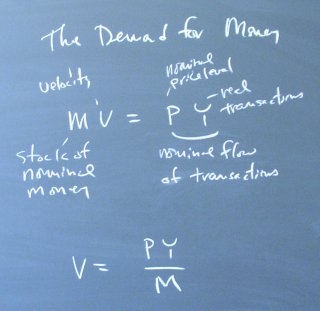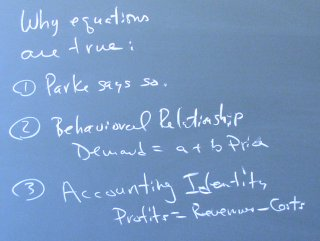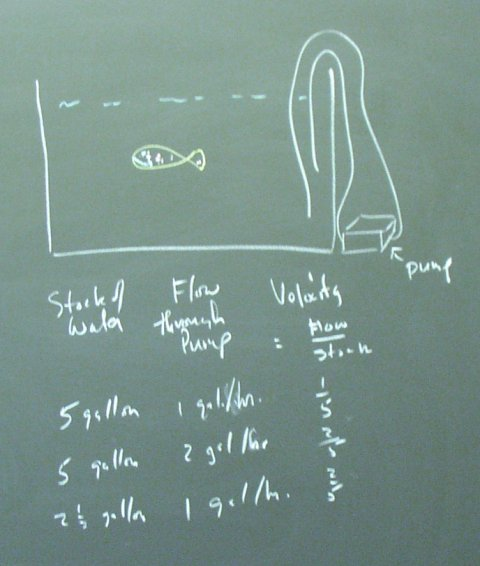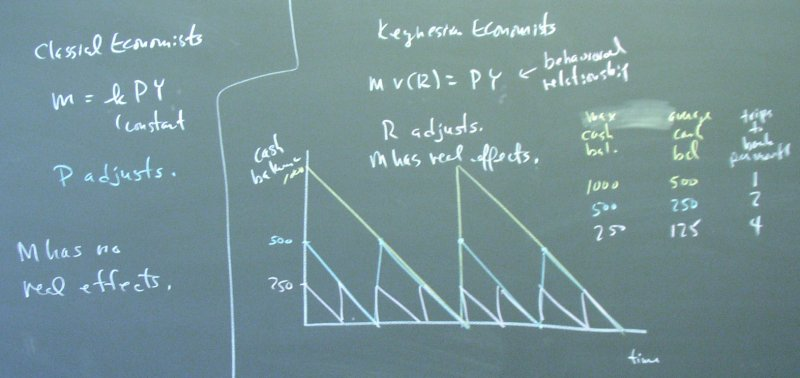## April 01, 2003

### The Demand for Money

Our discussion begins with a very important equation.This can be called the velocity equation or the equation of exchange.

We reviewed the reasons why equations are true.The velocity equation is true because it is an accounting identity. We observe the flow of transactions and the stock of money and define the ratio to be the velocity.

To understand velocity, we studied the flow and stock of water for a fish tank.The classical economists thought that velocity was constant, and the price level adjusted to accommodate the supply of money, which at the time was often gold coins.The Keynesians introduced the idea that the velocity of money depends on the interest, turning our accounting identity into a behavioral relation. If the veloicity depends on the interest rate, then changing the supply of money can force a change in the interest rate.

Here, we see a classic depiction of how the interest rate might affect the number of time per month that an agents travels to a bank to collect interest on his cash balances. This is the "shoe leather" explanation for why the velocity depends on the interest rate.

Posted by bparke at April 1, 2003 03:18 PM